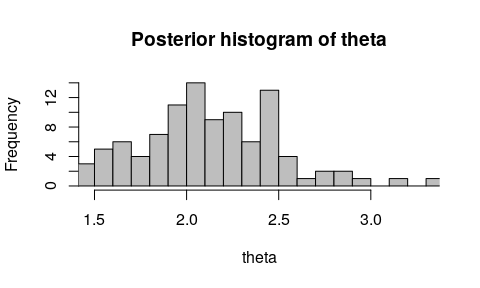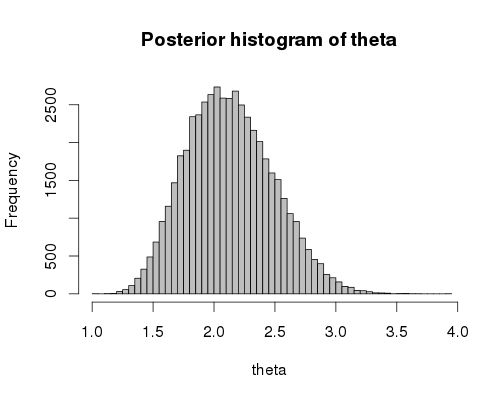# Using Coala for Approximate Bayesian Computation

#### coala 0.5.3

Approximate Bayesian Computation (ABC) is a simulation based method for Bayesian inference. It is commonly used in evolutionary biology to estimate parameters of demographic models. Coala makes it easy to conduct the simulations for an ABC analysis and works well together with the abc package for doing the estimation. To demonstrate the principle, we will estimate the parameter of an over-simplified toy model.

Let’s assume that we have 50 genetic loci from 10 individuals from a panmictic population. We will use the site frequency spectrum of the data as a set of summary statistics to estimate the scaled mutation rate, theta. Let’s assume we get the following frequency spectrum from the data:

sfs <- c(112, 57, 24, 34, 16, 29, 8, 10, 15)

We can now use coala to set the model up:

library(coala)
model <- coal_model(10, 50) +
feat_mutation(par_prior("theta", runif(1, 1, 5))) +
sumstat_sfs()

Note that we used par_prior to set a uniform prior between 1 and 5 for theta.

We can now easily simulate the model:

sim_data <- simulate(model, nsim = 2000, seed = 17)

For this toy model, we ran just 2000 simulations (to keep the time for building this document within a reasonable range). A real analysis will need many more, but you can reduce the simulation time by parallelizing them with the cores argument.

We need to prepare the simulation data for the abc package. We can use the create_abc_param and create_abc_sumstat functions for this purpose:

# Getting the parameters
sim_param <- create_abc_param(sim_data, model)
head(sim_param, n = 3)
##      theta
## 1 1.620203
## 2 1.216988
## 3 2.410258
# Getting the summary statistics
sim_sumstat <- create_abc_sumstat(sim_data, model)
head(sim_sumstat, n = 3)
##      sfs1 sfs2 sfs3 sfs4 sfs5 sfs6 sfs7 sfs8 sfs9
## [1,]   74   54   32   30   13   14   10    6    2
## [2,]   63   30   12   12   12   12   11    8    7
## [3,]  121   49   45   36   12   28   12    7    8

Now, we can estimate the posterior distribution of theta:

suppressPackageStartupMessages(library(abc))
posterior <- abc(sfs, sim_param, sim_sumstat, 0.05, method = "rejection")
hist(posterior, breaks = 20)Due to the low number of simulations, this posterior should only be treated as a rough approximation. We have prepared a version using a million simulations instead. Generating it took significantly longer (about two hours on a modern laptop), but we get a smoother estimate of the posterior distribution: